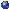## Events

##### AnalysisLaurent Lafleche, RLM 10.176: Semiclassical limit from the Hartree equation to the Vlasov-Poisson system
Wednesday, November 13, 2019, 01:00pm - 02:00pm
The Hartree equation is the mean field equation which describesthe evolution of a system of particles in interaction in quantummechanics. It can be proved that it converges in some weak senseto the Vlasov equation when the Planck constant \hbar becomesnegligible. In this talk, I will present how this convergencecan be quantitatively measured in the case of singular nonlinearinteractions such as the Coulomb interaction. To reach this goal,I will introduce the Wigner transform, a semiclassical versionof the Wasserstein-Monge-Kantorovitch distance introduced byF. Golse and T. Paul, and also a semiclassical analogue of thekinetic Lebesgue norms. One of the key step to reach this resultis the propagation in time of semiclassical moments, in the spiritof the proof of existence for the Vlasov-Poisson equation byP.-L. Lions and B. Perthame, and weighted Schatten norms of thesolution, which implies the boundedness of the spatial densityof particles. This can be proved by using the formal analogiesbetween the density operator formulation of quantum mechanicsand kinetic theory.Location: RLM 10.176